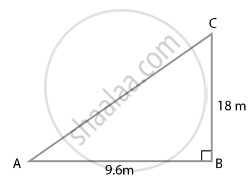# A flag pole 18 m high casts a shadow 9.6 m long. Find the distance of the top of the pole from the far end of the shadow. - Mathematics

Sum

A flag pole 18 m high casts a shadow 9.6 m long. Find the distance of the top of the pole from the far end of the shadow.

#### SolutionLet MN = 18 m be the flag pole and its shadow be LM = 9.6 m.

The distance of the top of the pole, N from the far end, L of the shadow is LN.

In right-angled ∆LMN,

LN2 = LM2 + MN2  ......[By Pythagoras theorem]

⇒ LN2 = (9.6)2 + (18)2

⇒ LN2 = 9.216 + 324

⇒ LN2 = 416.16

∴ LN = sqrt(416.16) = 20.4 m

Hence, the required distance is 20.4 m

Concept: Application of Pythagoras Theorem in Acute Angle and Obtuse Angle
Is there an error in this question or solution?

#### APPEARS IN

NCERT Mathematics Exemplar Class 10
Chapter 6 Triangles
Exercise 6.4 | Q 7 | Page 74

Share